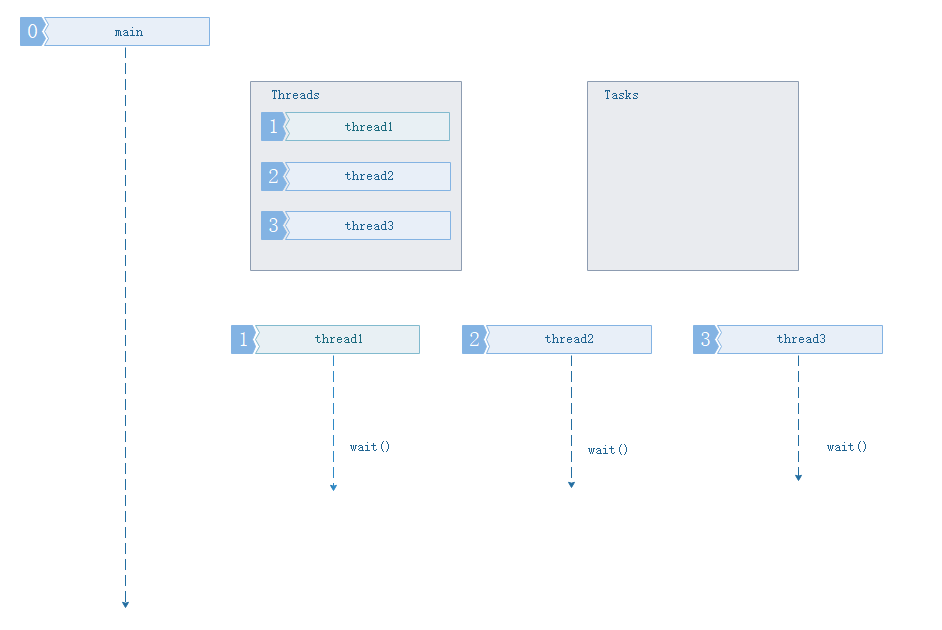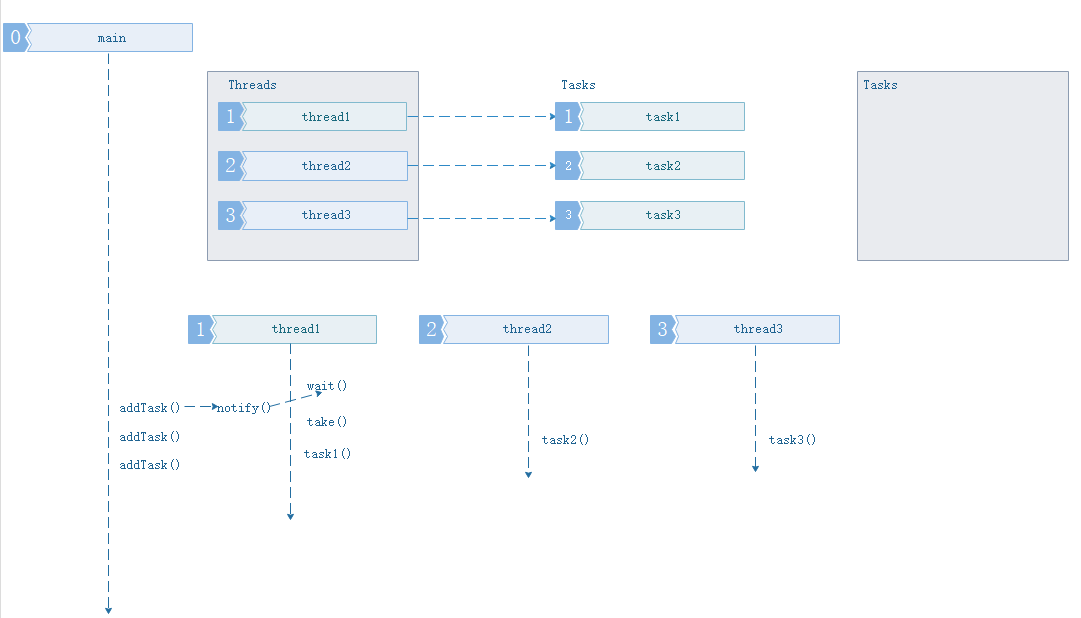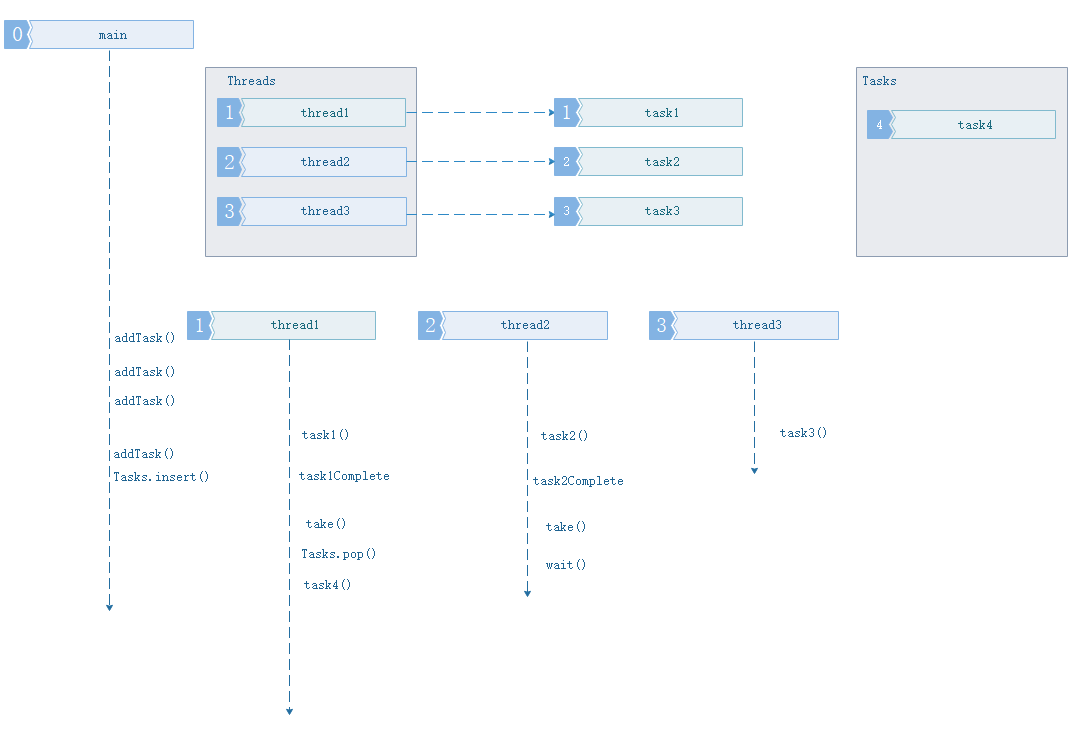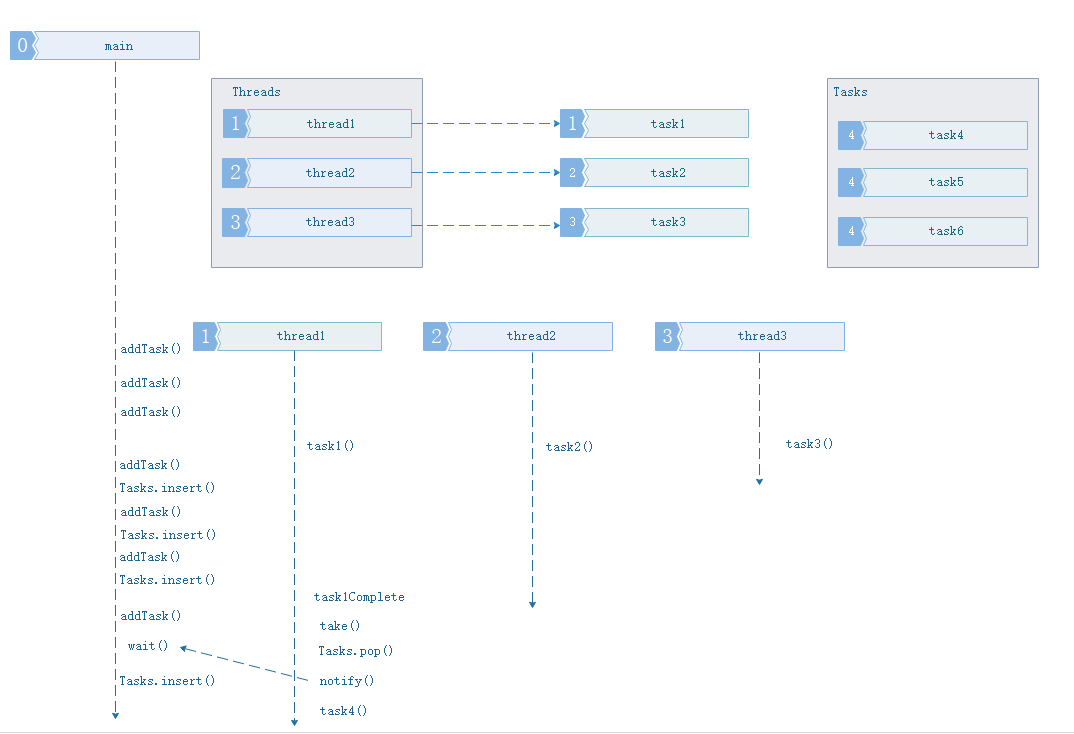• c++ 实现的线程池工程，可直接使用，其中线程数目可根据空闲情况自增减，并且实现线程跟任务无关联。
• 2：可以使用类成员函数/全局函数单独的为线程池添加一个任务,可以带多个参数。 3：线程池的线程数量可手动扩展，稍作修改可以修改为自动扩充。 是否下载可前往...
• 本文实例讲述了c++线程池实现方法。分享给大家供大家参考。具体分析如下： 下面这个线程池是我在工作中用到过的，原理还是建立一个任务队列，让多个线程互斥的在队列中取出任务，然后执行，显然，队列是要加锁的 ...
• ## C++线程池

万次阅读 2019-05-18 19:53:33
C++线程池 参考链接： 基于C++11实现线程池的工作原理;c++简单线程池实现 基础概念 线程池: 当进行并行的任务作业操作时，线程的建立与销毁的开销是，阻碍性能进步的关键，因此线程池，由此产生。使用多个线程，...

2019-05-17 20:16:52 原文链接

C++线程池
参考链接： 基于C++11实现线程池的工作原理;c++简单线程池实现
基础概念
线程池: 当进行并行的任务作业操作时，线程的建立与销毁的开销是，阻碍性能进步的关键，因此线程池，由此产生。使用多个线程，无限制循环等待队列，进行计算和操作。帮助快速降低和减少性能损耗。
线程池的组成
线程池管理器：初始化和创建线程，启动和停止线程，调配任务；管理线程池工作线程：线程池中等待并执行分配的任务任务接口：添加任务的接口，以提供工作线程调度任务的执行。任务队列：用于存放没有处理的任务，提供一种缓冲机制，同时具有调度功能，高优先级的任务放在队列前面
线程池工作的四种情况
1. 没有任务要执行，缓冲队列为空2. 队列中任务数量，小于等于线程池中线程任务数量3. 任务数量大于线程池数量,缓冲队列未满4. 任务数量大于线程池数量，缓冲队列已满线程池的C++实现
线程池的主要组成有上面三个部分：
队列
我们使用队列来存储工作，因为它是更合理的数据结构。 我们希望以与发送它相同的顺序启动工作。 但是，这个队列有点特殊。正如我在上一节中所说的，线程是连续的（好吧，不是真的，但我们假设它们是）查询队列要求工作。当有可用的工作时，线程从队列中获取工作并执行它。如果两个线程试图同时执行相同的工作会发生什么？ 好吧，程序会崩溃。 为了避免这种问题，我在标准C ++ Queue上实现了一个包装器，它使用mutex来限制并发访问。 让我们看一下SafeQueue类的一小部分示例：
void enqueue(T& t) {
std::unique_lock<std::mutex> lock(m_mutex);
m_queue.push(t);
}

要排队我们做的第一件事就是锁定互斥锁以确保没有其他人正在访问该资源。然后，我们将元素推送到队列中。 当锁超出范围时，它会自动释放。好吗，对吧？这样，我们使Queue线程安全，因此我们不必担心许多线程在相同的“时间”访问和/或修改它。
提交函数
线程池最重要的方法是负责向队列添加工作的方法。我打电话给这个方法提交。不难理解它是如何工作的，但它的实现起初可能看起来很吓人。让我们考虑应该做什么，之后我们会担心如何做到这一点。 什么：
接受任何参数的任何函数。立即返回“东西”以避免阻塞主线程。 此返回的对象最终应包含操作的结果。 完整的提交函数如下所示：
// Submit a function to be executed asynchronously by the pool template<typename F, typename...Args>

auto submit(F&& f, Args&&... args) -> std::future<decltype(f(args...))> {
// Create a function with bounded parameters ready to execute
std::function<decltype(f(args...))()> func = std::bind(std::forward<F>(f), std::forward<Args>(args)...);
// Encapsulate it into a shared ptr in order to be able to copy construct / assign

// Wrap packaged task into void function
};

// Enqueue generic wrapper function
m_queue.enqueue(wrapperfunc);

// Wake up one thread if its waiting
m_conditional_lock.notify_one();

// Return future from promise
}


队列完整源代码
// SafeQueue.h

#pragma once

#include <mutex>
#include <queue>

// Thread safe implementation of a Queue using a std::queue
template <typename T>
class SafeQueue {
private:
std::queue<T> m_queue; //利用模板函数构造队列

std::mutex m_mutex;//访问互斥信号量

public:
SafeQueue() { //空构造函数

}

SafeQueue(SafeQueue& other) {//拷贝构造函数

//TODO:
}

~SafeQueue() { //析构函数

}

bool empty() {  //队列是否为空

std::unique_lock<std::mutex> lock(m_mutex); //互斥信号变量加锁，防止m_queue被改变

return m_queue.empty();
}

int size() {
std::unique_lock<std::mutex> lock(m_mutex); //互斥信号变量加锁，防止m_queue被改变

return m_queue.size();
}
//队列添加元素

void enqueue(T& t) {
std::unique_lock<std::mutex> lock(m_mutex);
m_queue.push(t);
}
//队列取出元素

bool dequeue(T& t) {
std::unique_lock<std::mutex> lock(m_mutex); //队列加锁

if (m_queue.empty()) {
return false;
}
t = std::move(m_queue.front()); //取出队首元素，返回队首元素值，并进行右值引用

m_queue.pop(); //弹出入队的第一个元素

return true;
}
};

线程池完整代码
//ThreadPool.h

#pragma once

#include <functional>
#include <future>
#include <mutex>
#include <queue>
#include <utility>
#include <vector>

#include "SafeQueue.h"

private:

private:
int m_id; //工作id

public:
//构造函数

: m_pool(pool), m_id(id) {
}
//重载()操作

void operator()() {
std::function<void()> func; //定义基础函数类func

bool dequeued; //是否正在取出队列中元素

//判断线程池是否关闭，没有关闭，循环提取

while (!m_pool->m_shutdown) {
{
//为线程环境锁加锁，互访问工作线程的休眠和唤醒

std::unique_lock<std::mutex> lock(m_pool->m_conditional_mutex);
//如果任务队列为空，阻塞当前线程

if (m_pool->m_queue.empty()) {
m_pool->m_conditional_lock.wait(lock); //等待条件变量通知，开启线程

}
//取出任务队列中的元素

dequeued = m_pool->m_queue.dequeue(func);
}
//如果成功取出，执行工作函数

if (dequeued) {
func();
}
}
}
};

bool m_shutdown; //线程池是否关闭

SafeQueue<std::function<void()>> m_queue;//执行函数安全队列，即任务队列

std::mutex m_conditional_mutex;//线程休眠锁互斥变量

std::condition_variable m_conditional_lock; //线程环境锁，让线程可以处于休眠或者唤醒状态

public:
//线程池构造函数

}

void init() {
for (int i = 0; i < m_threads.size(); ++i) {

}
}

// Waits until threads finish their current task and shutdowns the pool

void shutdown() {
m_shutdown = true;
m_conditional_lock.notify_all(); //通知 唤醒所有工作线程

for (int i = 0; i < m_threads.size(); ++i) {

}
}
}

// Submit a function to be executed asynchronously by the pool
//线程的主要工作函数，使用了后置返回类型，自动判断函数返回值

template<typename F, typename...Args>
auto submit(F&& f, Args&&... args) -> std::future<decltype(f(args...))> {
// Create a function with bounded parameters ready to execute
//

std::function<decltype(f(args...))()> func = std::bind(std::forward<F>(f), std::forward<Args>(args)...);//连接函数和参数定义，特殊函数类型,避免左、右值错误

// Encapsulate it into a shared ptr in order to be able to copy construct // assign
//封装获取任务对象，方便另外一个线程查看结果

// Wrap packaged task into void function
//利用正则表达式，返回一个函数对象

};

// 队列通用安全封包函数，并压入安全队列

m_queue.enqueue(wrapper_func);

// 唤醒一个等待中的线程

m_conditional_lock.notify_one();

// 返回先前注册的任务指针

}
};


使用样例代码 参考连接： std::random_device;std::mt19937;std::uniform_int_distribution;;
#include <iostream>
#include <random>

std::random_device rd; //真实随机数产生器

std::mt19937 mt(rd()); //生成计算随机数mt;

std::uniform_int_distribution<int> dist(-1000, 1000);//生成-1000到1000之间的离散均匀分部数

auto rnd = std::bind(dist, mt);

//设置线程睡眠时间

void simulate_hard_computation() {
}

// 添加两个数字的简单函数并打印结果

void multiply(const int a, const int b) {
simulate_hard_computation();
const int res = a * b;
std::cout << a << " * " << b << " = " << res << std::endl;
}

//添加并输出结果

void multiply_output(int & out, const int a, const int b) {
simulate_hard_computation();
out = a * b;
std::cout << a << " * " << b << " = " << out << std::endl;
}

// 结果返回

int multiply_return(const int a, const int b) {
simulate_hard_computation();
const int res = a * b;
std::cout << a << " * " << b << " = " << res << std::endl;
return res;
}

void example() {
// 创建3个线程的线程池

// 初始化线程池

pool.init();

// 提交乘法操作，总共30个

for (int i = 1; i < 3; ++i) {
for (int j = 1; j < 10; ++j) {
pool.submit(multiply, i, j);
}
}

// 使用ref传递的输出参数提交函数

int output_ref;
auto future1 = pool.submit(multiply_output, std::ref(output_ref), 5, 6);

// 等待乘法输出完成

future1.get();
std::cout << "Last operation result is equals to " << output_ref << std::endl;

// 使用return参数提交函数

auto future2 = pool.submit(multiply_return, 5, 3);

// 等待乘法输出完成

int res = future2.get();
std::cout << "Last operation result is equals to " << res << std::endl;

//关闭线程池
pool.shutdown();
}

相关代码位置
展开全文后台开发 并行编程
• ## C++线程池实例

热门讨论 2014-01-07 14:04:01
一个c++线程池类的使用。包含一个线程池类，工程使用vc6.0编译器，整个程序演示了怎么使用一个线程池。
• c++一个很好的线程池demo，可以直接拿来用，效率很高。
• ## C++线程池

千次阅读 2019-01-21 21:01:24
1.概述：什么是线程池 因为程序边运行边创建线程是比较耗时的，所以我们通过池化的思想：在程序开始运行前创建多个线 程，这样，程序在运行时，只需要从线程池中拿来用就可以了．大大提高了程序运行效率。 2.如何...
复制这位同学：https://blog.csdn.net/liushengxi_root/article/details/83932654
1.概述：什么是线程池
因为程序边运行边创建线程是比较耗时的，所以我们通过池化的思想：在程序开始运行前创建多个线 程，这样，程序在运行时，只需要从线程池中拿来用就可以了．大大提高了程序运行效率。
2.如何实现线程池
一般线程池都会有以下几个部分构成：
总的来讲，就是先创建几个线程，然后这些线程等待任务队列，不为空拿出任务执行即可（任务可以是对象，也可以是某个函数）
3.实现：采用C++模板
#pragma once

#include "UtilsLib.h"
//----------------------------------------------------------------------------
//----------------------------------------------------------------------------
template <typename T>
{
public:
public:
bool Stop();										// 停止线程池
private:
static void* Worker(void* arg);						// 工作线程，读取任务队列并执行
void Run();											// 运行

private:
std::mutex mQueueMutex;								// 任务队列的互斥量
std::condition_variable mCondVar;
bool mStoped;										// 线程池是否停止
};

//----------------------------------------------------------------------------
// 函数实现
//----------------------------------------------------------------------------
template <typename T>
mStoped(false)
{
for (int i = 0; i < num; i++)
{
}
}
//----------------------------------------------------------------------------
template <typename T>
{
if (mStoped == false)
Stop();
}
//----------------------------------------------------------------------------
template <typename T>
{
mQueueMutex.lock();
mQueueMutex.unlock();
mCondVar.notify_one();
return true;
}
//----------------------------------------------------------------------------
template <typename T>
{
pool->Run();
return pool;
}
//----------------------------------------------------------------------------
template <typename T>
{
while (!mStoped)
{
std::unique_lock<std::mutex> lock(this->mQueueMutex);
this->mCondVar.wait(lock, [this] {
});
// 如果任务队列不为空，就停下来等待唤醒
if (this->mStoped)
{
LOG(INFO) << "Thread Pool Run Function Return";
return;
}
{
continue;
}
else
{
{
LOG(INFO) << "Hava Run A Request!";
}
}
}
}
//----------------------------------------------------------------------------
template <typename T>
{
mStoped = true;
mCondVar.notify_all();
for (auto& w : mWorkers)
{
w.join();
}
LOG(INFO) << "All Thread Release !!!";
return true;
}
//----------------------------------------------------------------------------
#endif

class Task
{
public:
typedef void(*pFunc)(void*);
Task(pFunc pf, void* param) :mpParam(param), mpFun(pf) {};
inline void operator()()
{
// mpFun(mpParam);
}
void Run()
{
{
std::unique_lock<std::mutex> lock(mQueueMutex);
std::cout << "发送一个请求:" << *(int*)mpParam << std::endl;;
}

mpFun(mpParam);
}
void* mpParam;
pFunc mpFun;
// 静态函数
static void Worker(void* param)
{
{
std::unique_lock<std::mutex> lock(mQueueMutex);
std::cout << "收到一个请求:" << *(int*)param <<
}

}
};

int main()
{
}

展开全文• C++线程池结合IOCP完成端口实现socket高并发服务端程序 包含mysql数据库操作、json数据解析
• 线程池2.线程池作用及应用场合3. 线程池实例 1.线程池 线程池是在应用程序启动之后，将立即创建一定数量的线程(N1)，放入空闲队列中。这些线程都是处于阻塞（Suspended）状态，不消耗CPU，但占用较小的内存空间。当...


文章目录

1.线程池
2.线程池作用及应用场合
3. 线程池实例

1.线程池

线程池是在应用程序启动之后，将立即创建一定数量的线程(N1)，放入空闲队列中。这些线程都是处于阻塞（Suspended）状态，不消耗CPU，但占用较小的内存空间。当任务到来后，缓冲池选择一个空闲线程，把任务传入此线程中运行。当N1个线程都在处理任务后，缓冲池自动创建一定数量的新线程，用于处理更多的任务。在任务执行完毕后线程也不退出，而是继续保持在池中等待下一次的任务。当系统比较空闲时，大部分线程都一直处于暂停状态，线程池自动销毁一部分线程，回收系统资源。

2.线程池作用及应用场合

作用：并发处理数量巨大但相对时间较短的任务，缩短传统线程方案中不停创建线程，销毁线程的时间。 目前的大多数网络服务器，包括Web服务器、Email服务器以及数据库服务器等都具有一个共同点，就是单位时间内必须处理数目巨大的连接请求，但处理时间却相对较短。 传统多线程方案中我们采用的服务器模型则是一旦接受到请求之后，即创建一个新的线程，由该线程执行任务。任务执行完毕后，线程退出，这就是是“即时创建，即时销毁”的策略。 尽管与创建进程相比，创建线程的时间已经大大的缩短，但是如果提交给线程的任务是执行时间较短，而且执行次数极其频繁，那么

展开全文线程池实例
github链接 在学习多线程的时候必不可少的一个知识点就是线程池，在web服务器中我们就常常会遇见它。在c++中没有标准的线程池，但这个轮子其实早就被大牛们造好了，现在我们就来看看这个github高星线程池的实现。
接口

public:

template <class F, class... Args>
auto enqueue(F &&f, Args &&... args)
-> std::future<typename std::result_of<F(Args...)>::type>;

private:
// need to keep track of threads so we can join them

// synchronization
std::mutex queue_mutex;
std::condition_variable condition;
bool stop;
};


// the constructor just launches some amount of workers
for (size_t i = 0; i < threads; ++i)
workers.emplace_back([this] {
for (;;) {

{
/* 注意这里是一个lockguard*/
std::unique_lock<std::mutex> lock(this->queue_mutex);
this->condition.wait(
lock, [this] { return this->stop || !this->tasks.empty(); });
/* 醒来以后看是否需要停止，如果任务队列非空！
*可以发现它的处理中必须将任务队列所有的任务处理完才退出 */
return;
/* 从任务队列pop front,执行任务 */
}
}
});
}

// the destructor joins all threads
{
std::unique_lock<std::mutex> lock(queue_mutex);
stop = true;
}
condition.notify_all();
/* 此时所有的任务必须全部做完才能退出 */
worker.join();
}


在添加任务的enqueue函数中会有一堆c++11并发有关的名词需要你去认识。
std::result_of获得一个函数的返回值std::forward保持参数的左右值属性，实现完美转发std::bind将函数通过适配器的方式绑定，生成一个新的可调用对象来“适应”原对象的参数列表。std::futurec++11的一种新同步机制中作为沟通桥梁，可配合std::promise,std::packaged_task,std::async等来实现多线程间函数异步调用的功能。std::packaged_task可传入一个可调用对象/函数和参数，并可以用get_future()来返回一个std::future对象,可以通过它来确定调用函数的返回值以表示函数“就绪”（已完成），实现“同步”。传入可以通过这个future.get()来获取执行函数的返回值，当然这个函数如果没有执行完毕，此时future.get()会阻塞等待。
首先我们可以看到enqueue的返回值是一个future,用户可以通过这个future.get()获得函数的返回值，保证任务函数已就绪。当然毕竟是个阻塞操作，我觉得服务器应该是不会轻易去调用这个get的。我们可以看到函数的返回值类型typename std::result_of<F(Args...)>::type>获得是任务函数F的返回值类型。如函数是int func(long,char)则获得的type是int,这符合我们的预期。
  auto task = std::make_shared<std::packaged_task<return_type()>>(
std::bind(std::forward<F>(f), std::forward<Args>(args)...))

接着我们用make_shared去获得一个传入函数和参数的packaged_task的智能指针。 再用packaged_task.get_future()获取std::future类型的res,并在enqueue函数最后作为返回值返回给用户。 然后再在临界区加锁并添加任务（又用lamada表达式，让packaged_task执行其任务函数,以lamada函数的形式去适配任务队列的类型std::function<void()>，std::queue<std::function<void()>> tasks），并用条件变量通知挂起的线程执行任务。
// add new work item to the pool
template <class F, class... Args>
auto ThreadPool::enqueue(F &&f, Args &&... args)
-> std::future<typename std::result_of<F(Args...)>::type> {
using return_type = typename std::result_of<F(Args...)>::type;

std::bind(std::forward<F>(f), std::forward<Args>(args)...));

{
std::unique_lock<std::mutex> lock(queue_mutex);

// don't allow enqueueing after stopping the pool
if (stop)

}
condition.notify_one();
return res;
}

到此线程池内部就已经一览无余了。
测试代码中,我们用results来存储每个任务返回的future,这些任务将会在线程池中的各个线程中执行,用户从个result.get()去获得这些函数的返回值，当然如果传入函数的返回值是void,那调用std::future<int>.get()也一样表示任务函数就绪。
#include "ThreadPool.h"
#include <chrono>
#include <iostream>
#include <vector>
int main() {

std::vector<std::future<int>> results;

for (int i = 0; i < 8; ++i) {
results.emplace_back(pool.enqueue([i] {
// std::cout << "hello " << i << std::endl;
std::cout << "begin:" << std::this_thread::get_id() << std::endl;
std::cout << "end" << std::this_thread::get_id() << std::endl;
// std::cout << "world " << i << std::endl;
return i * i;
}));
}
// std::cout<<"there"<<std::endl;

for (auto &&result : results) {
std::cout << "wait for the ret " << std::endl;
std::cout << result.get() << ' ' << std::endl;
// std::cout << std::endl;
}

/*   int x = 1;
auto res = pool.enqueue([](int &y) { y = 99; }, std::ref(x));
//auto res = pool.enqueue([](int &y) { y = 99; }, x);
res.get();
std::cout<<x<<std::endl;
return 0;
*/}

可以看到上方有我注释掉的一段代码，是和std::bind有关的一个坑，如果使用x和使用std::ref(x)是两种结果，这和std::bind的拷贝行为有关，需要用std::ref间接传入“左值”。 std::ref
源码
#ifndef THREAD_POOL_H

#include <condition_variable>
#include <functional>
#include <future>
#include <memory>
#include <mutex>
#include <queue>
#include <stdexcept>
#include <vector>

public:

template <class F, class... Args>
auto enqueue(F &&f, Args &&... args)
-> std::future<typename std::result_of<F(Args...)>::type>;

private:
// need to keep track of threads so we can join them

// synchronization
std::mutex queue_mutex;
std::condition_variable condition;
bool stop;
};

// the constructor just launches some amount of workers
for (size_t i = 0; i < threads; ++i)
workers.emplace_back([this] {
for (;;) {

{
std::unique_lock<std::mutex> lock(this->queue_mutex);
/* 注意这里是一个lockguard*/
this->condition.wait(
lock, [this] { return this->stop || !this->tasks.empty(); });
/* 醒来以后看是否需要停止，如果任务队列非空！
*可以发现它的处理中必须将任务队列所有的任务处理完才退出 */
return;
/* 从任务队列pop front,执行任务 */
}
}
});
}

// add new work item to the pool
template <class F, class... Args>
auto ThreadPool::enqueue(F &&f, Args &&... args)
-> std::future<typename std::result_of<F(Args...)>::type> {
using return_type = typename std::result_of<F(Args...)>::type;

std::bind(std::forward<F>(f), std::forward<Args>(args)...));

{
std::unique_lock<std::mutex> lock(queue_mutex);

// don't allow enqueueing after stopping the pool
if (stop)

}
condition.notify_one();
return res;
}

// the destructor joins all threads
{
std::unique_lock<std::mutex> lock(queue_mutex);
stop = true;
}
condition.notify_all();
/* 此时所有的任务必须全部做完才能退出 */
worker.join();
}

#endif


展开全文多线程
• NULL 博文链接：https://songpengfei.iteye.com/blog/1180345
• 由于c++标准库没有提供线程池，但是很多时候，我们需要使用线程池来执行任务从而提高效率，这也避免了频繁的启动、终止线程所带来的损耗。 设计 由于C++在不同平台有不同的线程实现，因此，为了保证跨平台化与通用...多线程
• 思路：创建线程池时启动固定个数线程，线程函数中循环监听任务队列，取出任务并执行，在此处我将指针函数作为任务传递入口，存在弊端就是任务处理函数签名固定了，灵活性低。当然，也可以将任务进行封装，使用C++的...多线程 队列 queue
• 1. 线程池原理 在传统服务器结构中，常用一个总的监听线程监听新用户连接，当有一个新用户进入时，服务器就开启一个新的线程，用于处理这个用户的数据收发，这个线程只服务于这个用户，当用户与服务器端连接关闭...多线程 并发编程 队列
• 线程池原理介绍 线程池是一种多线程处理形式，处理过程中将任务添加到队列，然后在创建线程后自动启动这些任务。线程池线程都是后台线程。每个线程都使用默认的堆栈大小，以默认的优先级运行，并处于多线程单元中。...C
• 一、线程池的概念 线程开的过多会带来调度开销，进而影响缓存局部性和整体性能。而线程池维护这多个线程，等待着监督管理制者分配可并发执行任务。这避免了在处理短时间任务时创建与销毁线程的代价。线程池能保证...
• 线程池是一种多线程处理形式，将任务添加到队列，创建线程后自动启动这些任务 降低资源消耗。线程池可以避免频繁的创建线程和销毁线程，线程池中线程可以重复使用 提高响应速度。线程池省去线程创建的这段时间 ...多线程
• 线程池的任务就在于负责这些线程的创建，销毁和任务处理参数传递、唤醒和等待。1、创建若干线程，置入线程池。2、任务达到时，从线程池取空闲线程。3、取得了空闲线程，立即进行任务处理。4、否则新建一个线程，并......

# c++线程池c++ 订阅